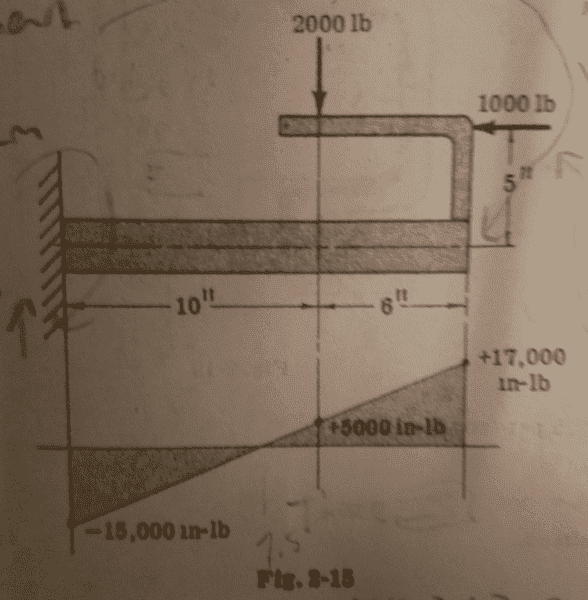# Bending moment diagram equation method

## Homework Statement

Draw the bending moment diagramthe top part is the question, the bottom part is the answer.

## The Attempt at a Solution

There is a force at the wall in x and y direction and a moment ##F_x, F_y, M_w##
Sum of forces in x : ##F_x-1000=0, F_x=1000##
Sum of forces in y : ##F_y-2000=0, F_y=2000##
Sum of moments at wall, clockwise = positive : ##M_w+2000*10-5000=0,M_x=-15000##

So... I am not sure how to write the equations for moment here. I only managed to come up with this equation :
##M_x+2000(10-x)-1000*5=0## ---> ##M_x=-15000+2000x##
This equation... it works out for the entire beam... but it has to be wrong. I originally had 2 equations, one for the left and right side of the downward 2000 force, but when I realized the first equation covered the whole beam I dropped it.

I think the shear diagram is a 2000lb constant line from x=0 to x=16, so the moment diagram is the integral of that M_w+area under shear curve ##=-15000+2000*16=17000##, which is correct according to the given answer...

But how do I do this with an equation? I hate the graphical method.

haruspex
Homework Helper
Gold Member
2020 Award
how do I do this with an equation? I hate the graphical method.
The main beam does not "know" about the two applied forces, except via their effects at its right hand end. So replace them by appropriate forces and moments applied there.

•fahraynk
Chestermiller
Mentor
In my judgment, your original analysis was correct. Beyond x=10, the moment is constant at 5000 in-lb.

•fahraynk
haruspex
Homework Helper
Gold Member
2020 Award
In my judgment, your original analysis was correct. Beyond x=10, the moment is constant at 5000 in-lb.
Break the system into two components: the horizontal main beam and L-shaped bracket.
Where they meet, the forces on the bracket must be 2000lb up, 1000lb to the right, and a clockwise moment of 17000 in-lb.
Correspondingly, at that end, the main beam is subject to 2000lb down, 1000lb to the left, and an anticlockwise moment of 17000 in-lb.

•fahraynk
@Chestermiller constant after x=10? I thought I needed a second equation where instead of 2000(10-X) it would become 2000(X-10)

Last edited:
@haruspex Say I do what you said and I pretend the beam isnt there and just write it as a force on the beam at the end... How should I write the equation? I'm still confused... Do I or do I not add the moments into the equation... (M_E=moment at right end of beam, F_Y is reactionary force on left side of beam in y direction=2000Lb))##-M_x+F_Y*x-M_E+2000(16-x)##-->##-15000-17000+2000(16)##
I am fundamentally confused. (did not add horizontal forces because assuming they are in plane of beam)

haruspex
Homework Helper
Gold Member
2020 Award
when I write an equation for the moment, I should not add the components from the top beam unless I add them at a distance of x=16
Maybe... Not quite sure what you mean there, so let me be quite clear.
For the sake of illustration, suppose the beam continues past 16 in, i.e. out past the junction with the angled bracket.
If the vertical 2000lb were applied directly to the main beam then at a point x from the left you would count its moment as:
- 0 for x < 10 in
- 2000 (x-10) for x > 10 in.
With the 2000 lb as in the diagram:
- 0 for x < 16 in
- 2000 (x-10) for x > 16 in
Only the threshold value of x at which that moment is included changes. The numerical value of the moment when it is included does not change.

The method of breaking it into two components gets the same result by a slightly different method:
- 0 for x < 16 in
- 2000 (x-16) for x > 16 in
- plus a counterclockwise moment of 2000 (6) for x > 16

I prefer the component method because it also makes it clear what to do with the horizontal force on the bracket, namely:
- 0 for x < 16
- a counterclockwise moment of 1000 (5) for x > 16.

haruspex
Homework Helper
Gold Member
2020 Award
@haruspex Say I do what you said and I pretend the beam isnt there and just write it as a force on the beam at the end... How should I write the equation? I'm still confused... Do I or do I not add the moments into the equation... (M_E=moment at right end of beam, F_Y is reactionary force on left side of beam in y direction=2000Lb))##-M_x+F_Y*x-M_E+2000(16-x)##-->##-15000-17000+2000(16)##
I am fundamentally confused. (did not add horizontal forces because assuming they are in plane of beam)
My last post crossed with yours. I'll give you a chance to read mine to see if it clears the matter up.

•fahraynk
My last post crossed with yours. I'll give you a chance to read mine to see if it clears the matter up.
sorry late reply holiday weekend. Can you tell m
##M_X## is moment at left end of beam, M=moment at point in question
So... if I cut the beam in the middle ##M_X+2000x=M##
This 2000x is the force at the wall up. So I add the moment AND the force in y direction from the left side of the beam when I cut the beam down the middle? This works because it matches the moment diagram provided in the book.

This goes all the way to x=16. Now at x=16.... This equation actually still gives me the answer. ##-15000+(2000*16)=17000##
Is this because the 1000Lb force is considered to act at the beam, not at a distance 5" from the beam?

haruspex
•